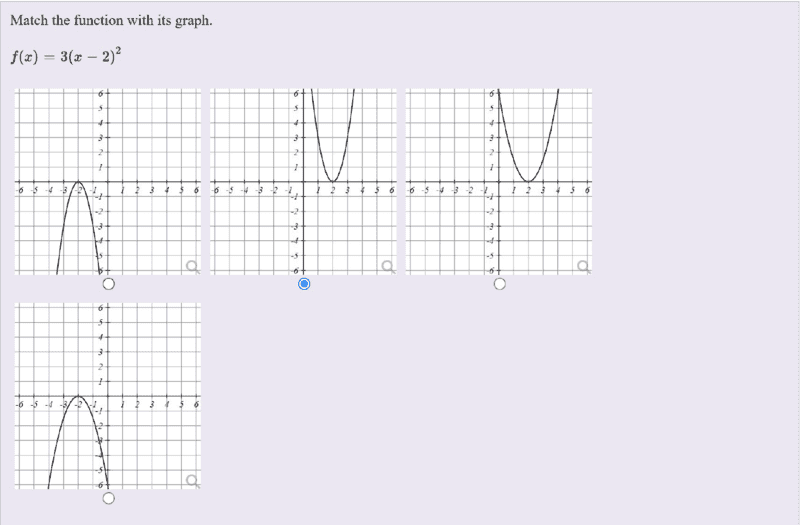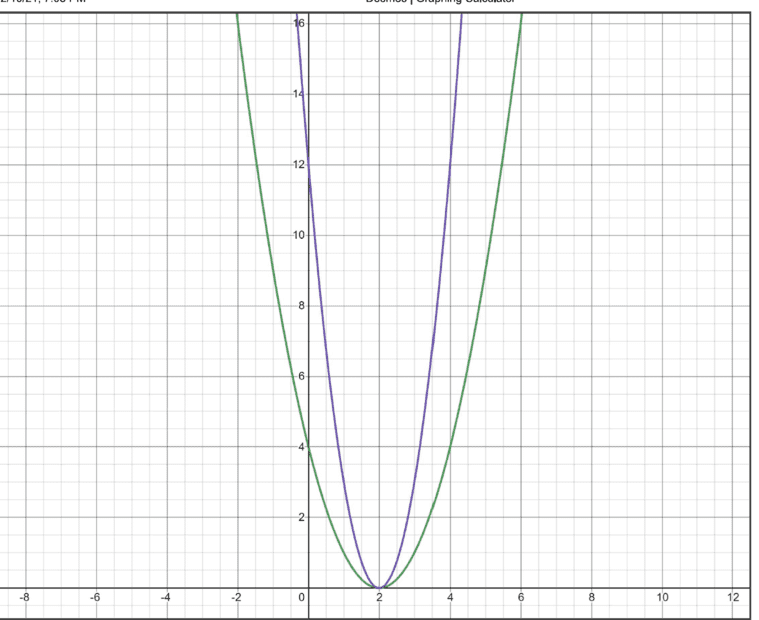# Solve the vertical stretch/compression graph problem

• chwala

#### chwala

Gold Member
Homework Statement
see attached
Relevant Equations
Vertical stretch/compression knowledge
This is the problem,Let ##y=f(x)= (x-2)^2##. The graph of ##y=af(x)##can be obtained from the graph of ##y=f(x)## by a stretch parallel to the y- axis with scale factor ##a##. In our case here, ##a=3##, therefore the corresponding graph is as indicated in blue. Find my graph below using desmos.Last edited:
You can check what the value of ##f(1)## is.

•chwala
##f(1) =1##, therefore ##af(1)=3f(1)= 3⋅1 =3##
From## f(x)## to ##af(x)##, the scale factor is ##k=3##

Last edited:
##f(1) =1##, therefore ##af(1)=3f(1)= 3.1 =3##
So indeed, the correct graph is the second one.

From## f(x)## to ##af(x)##, the scale factor is ##k=3##
That's correct, but I don't see how this is related to the question of finding the correct graph.

True, not related mate...cheers
So indeed, the correct graph is the second one.

That's correct, but I don't see how this is related to the question of finding the correct graph.

##f(1) =1##, therefore ##af(1)=3f(1)= 3.1 =3##
From## f(x)## to ##af(x)##, the scale factor is ##k=3##
You may want to write ##3 \!\cdot \!\!1## as
##3 \cdot 1 ##
rather than as
##3.1## .

You may want to write ##3 \!\cdot \!\!1## as
##3 \cdot 1 ##
rather than as
##3.1## .
Yes Sammy...actually I didn't forget, it is only that my android phone wasn't opening the tabs related to signs...i will for sure have that fixed.

•SammyS